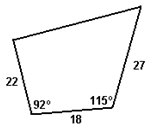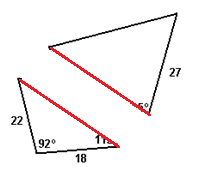# Trigonometry from a Geometric Perspective

3 teachers like this lesson
Print Lesson

## Objective

SWBAT use trigonometry to solve problems that are geometric in nature.

#### Big Idea

Use the Law of Sines, Law of Cosines, SAS Area Formula, and SOH CAH TOA to solve problems involving triangles.

## Launch and Explore

10 minutes

Over the last two days of class we learned three formulas (Law of Cosines, Law of Sines, Area = 1/2bcsinA). The worksheet that students will be working on today will give practice with these formulas. Since I do not specify which formula to use, students will get valuable practice identifying which formula(s) are appropriate to use with the given information. Some problems do not require these new formulas at all – they will be working with right triangles and can use SOH CAH TOA to set up their equations. This will be a good test to see if they can find the most efficient way to solve each problem.

Question #11 is often difficult for my students to complete. They have to find the area of the quadrilateral (shown below) and often have trouble knowing where to start or keeping track of the measurements they are finding.I am going to start class with this question and have them work on it with their table groups. I plan to give them about ten minutes to work on it. There are a few levels of scaffolding to help out students who are having trouble.

• If a student is completely stuck, refer them to the title of the worksheet (Triangle Problems) to see if that gives them an idea of how to divide the shape.
• If a student divides the quadrilateral into triangles and does not know what to use, have them draw the two triangles separately so that they can clearly see what information is given for each triangle and which one they should start with.• Organization is the most important part of this problem, so if students draw the triangles separately, they will be able to see what they do know about each triangle and they will be able to find the missing values.

## Share

10 minutes

After students have a sufficient amount of time to work on Question #11, choose a student or ask for a volunteer to share their work. I will have the student show their organized work or have them write it out in a systematic way. You want the students to be able to easily follow along. Students will have to do about 4 different formulas for this problem, so it is good if the work is organized so that they can pinpoint where they went wrong if they did not get the correct answer.

A good conversation to have about this problem is how rounding can make a difference in the answer. Choose a student who rounded very differently than the student who shared their work and see how it affects the final answer. Rounding to the nearest tenth will produce a substantial difference than someone who rounds to the nearest thousandth. Develop some class norms about what rounding is appropriate. Getting everyone on the same page will make it easier to have common conversations about these problems.

## Group Work

30 minutes

After working on Question #11 together, students can use the rest of the hour to continue to work on this with their groups. In the video below I talk about another problem on the worksheet that will be challenging for students.

When students leave today, I want to have a sense of what they did well and what they still need help with. A good idea is to walk around class with a clipboard and take notes on those things. When we start class tomorrow we can go over the things that went well and things that they still need work on.

When I teach this lesson, I often find that students are using the Law of Sines or Law of Cosines with right triangles. While they are getting the correct answers, it is more efficient to use SOH-CAH-TOA. A good way to end class would be to make a list of the different "Triangle Tools" and think of when we use each one.